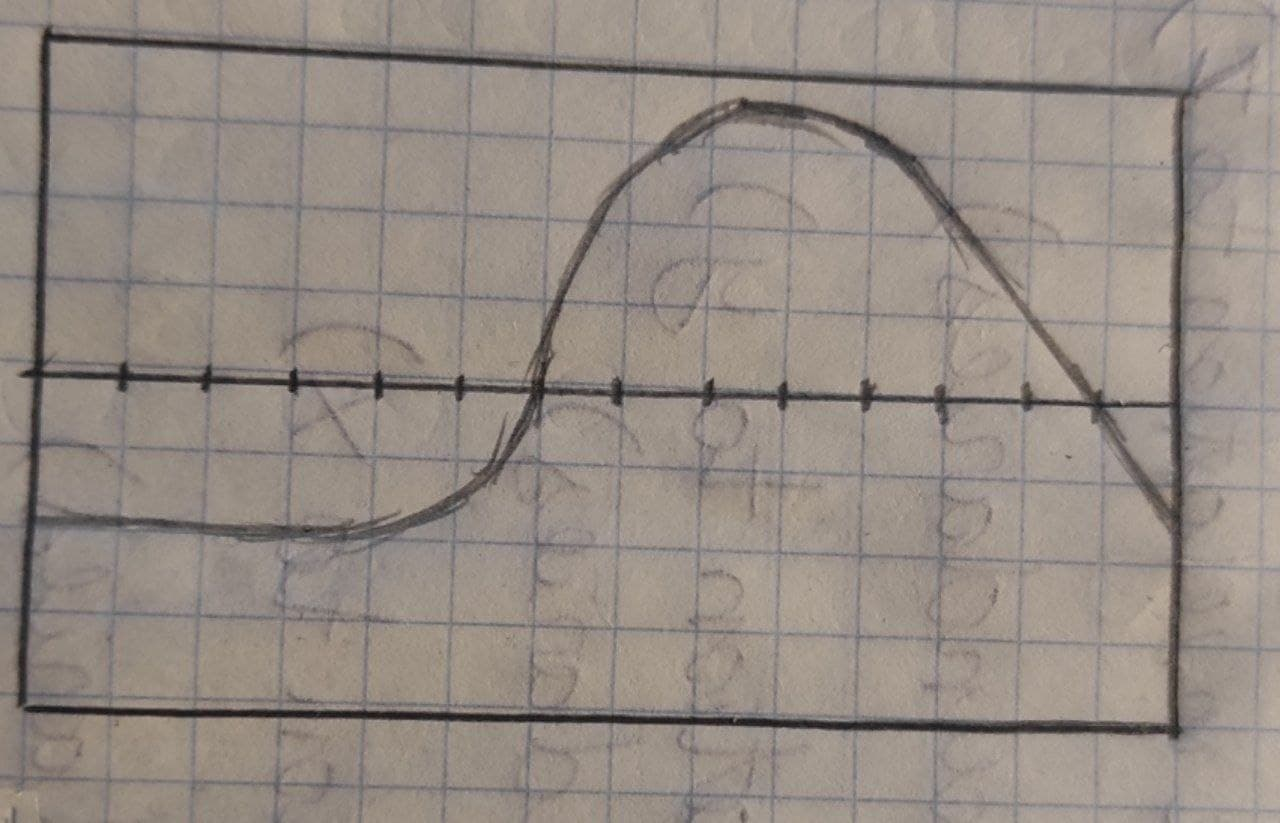# Do the following for the given curve on the given interval. a. Set up an integral forpancha3 2021-09-11 Answered
Do the following for the given curve on the given interval.
a. Set up an integral for the length of the curve.
b. Graph the curve to see what it looks like.
c. Use your​ graphers
You can still ask an expert for help

• Live experts 24/7
• Questions are typically answered in as fast as 30 minutes
• Personalized clear answers

Solve your problem for the price of one coffee

• Math expert for every subject
• Pay only if we can solve itMacsen Nixon

$\frac{dy}{dx}=\frac{d}{dx}\left(\mathrm{sin}\left\{x\right\}-\mathrm{cos}\left\{x\right\}\right)$
$=\mathrm{cos}\left\{x\right\}-\left[x\left(-\mathrm{sin}\left\{x\right\}\right)+\mathrm{cos}\left\{x\right\}\right]$
$=\mathrm{cos}\left\{x\right\}+2\mathrm{sin}\left\{x\right\}+\mathrm{cos}\left\{x\right\}$
$=2\mathrm{cos}\left\{x\right\}+x\mathrm{sin}\left\{x\right\}$
a) set up integral
$L={\int }_{0}^{2\pi }{\sqrt{\left(1+\frac{dx}{dy}\right\}}}^{2}dx$
$={\int }_{0}^{2\pi }\sqrt{{\left(1+2\mathrm{cos}\left\{x\right\}+\left\{x\right\}\mathrm{sin}\left\{x\right\}\right)}^{2}}dx$
$={\int }_{0}^{2\pi }\sqrt{1+4\mathrm{cos}\left\{x\right\}+{x}^{2}{\mathrm{sin}}^{2}x+4x\mathrm{cos}\left\{x\right\}\mathrm{sin}\left\{x\right\}}$
b)c) $\lambda ={\int }_{0}^{2\pi }\sqrt{1+{\left(\frac{dy}{dx}\right)}^{2}}$
$={\int }_{0}^{2\pi }\sqrt{1+{\left(2\mathrm{cos}\left\{x\right\}+x\mathrm{sin}\left\{x\right\}\right)}^{2}}dx$
$={\int }_{0}^{2\pi }\sqrt{1+4{\mathrm{cos}}^{2}\left\{x\right\}+{x}^{2}{\mathrm{sin}}^{2}\left\{x\right\}+4x\mathrm{cos}\left\{x\right\}\mathrm{sin}\left\{x\right\}}$
${\left[\frac{1}{2\sqrt{1+4{\mathrm{cos}}^{2}\left\{x\right\}+{x}^{2}<}}}_{}^{}$